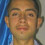## Tuesday, June 09, 2009

### How will WolframAlpha change math instruction?

When portable calculators were invented, we debated whether students should be allowed to use them in school and on tests. WolframAlpha takes this question beyond grade school.

Stephen Wolfram, co-founder of Wolfram Research, invented and commercialized Mathematica, an incredibly powerful symbolic math program. A command line version of Mathematica is embedded in Wolfram's latest product, the WolframAlpha Web service. Here are a few examples:

• Enter y = 10 x, and you are told that it is the equation for a straight line with x and y intercepts at 0 and a slope of 10, and the equation is plotted.
• Enter x^2, and you are told it is a parabola, which it plots and gives the global minimum, derivative and indefinite integral.
• Enter x^2 + y^2 + z^2= 10, and you get a plot of a sphere along with its center, radius, diameter, volume and surface area and other information including derivatives.
• Enter sin(x)^2 + cos(x)^2, and you get a plot of a constant 1 along with series representations and integrals.
• Enter derivative x^3, and you get the derivative and its plot plus an indefinite integral and global maximum.
• Enter integral x^2 + 3x dx, and you get the indefinite integral along with its plot. If you click on "show steps," you see the steps leading to the solution.
• Enter definite integral x^2 (0,1), and you get the definite integral from 0 to 1 along with a plot.
You get the idea -- try a few for yourself, and you will be amazed at the power of this symbolic math program.

Should students of algebra, geometry, trigonometry, finance, statistics, calculus, etc. be allowed to use WolframAlpha in class, for homework, during exams?

P. S. For links to further discussion, see this post.

#### 1 comment:

1.Larry press wrote: "Should students of algebra, geometry, trigonometry, finance, statistics, calculus, etc. be allowed to use WolframAlpha in class, for homework, during exams?"

I feel that students should be allowed to use WolframAlpha to do there assignments in class and at home, but should not use it on exams. Students should understand how to solve problems by hand first and than check there work with Wolfram. To me Wolfram is like a teacher that is there 24/7 telling the student if the answer they got is correct. Some books do have the answer in the back but not all. They either have all the answer for the even problems or the odd problems.

I don't favor WolframAlpha being used in exams because that would be considered cheating. A student should be able to do it on there own at the time of the exam.